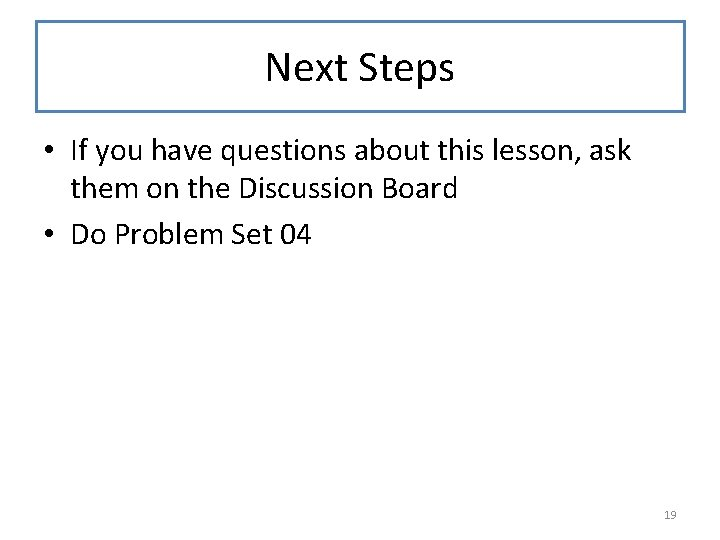# NonEmpty Lists CS 5010 Program Design Paradigms Bootcamp

• Slides: 19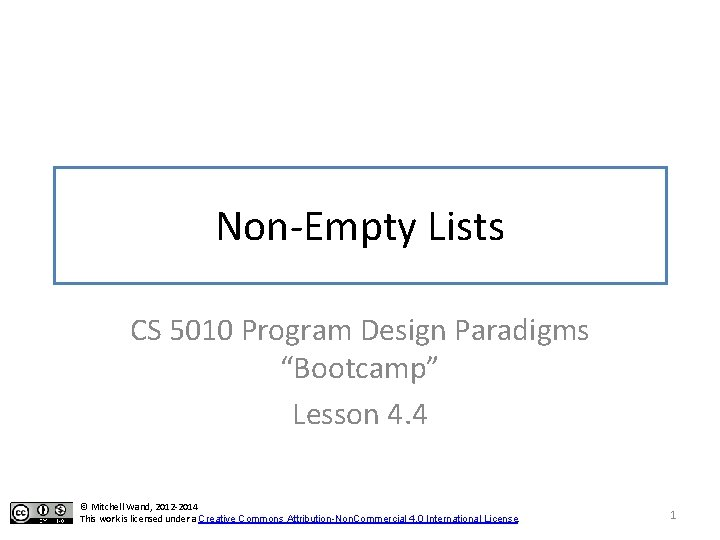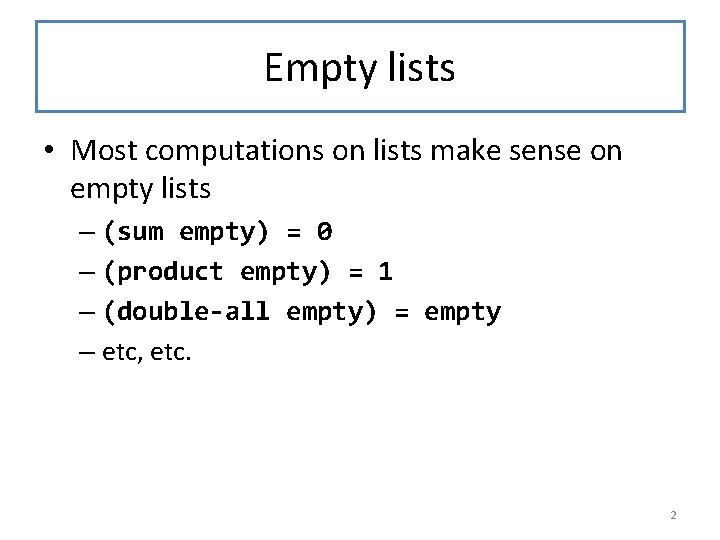Empty lists • Most computations on lists make sense on empty lists – (sum empty) = 0 – (product empty) = 1 – (double-all empty) = empty – etc, etc. 2Non-empty lists • But some computations don't make sense for empty lists – min, max – average 3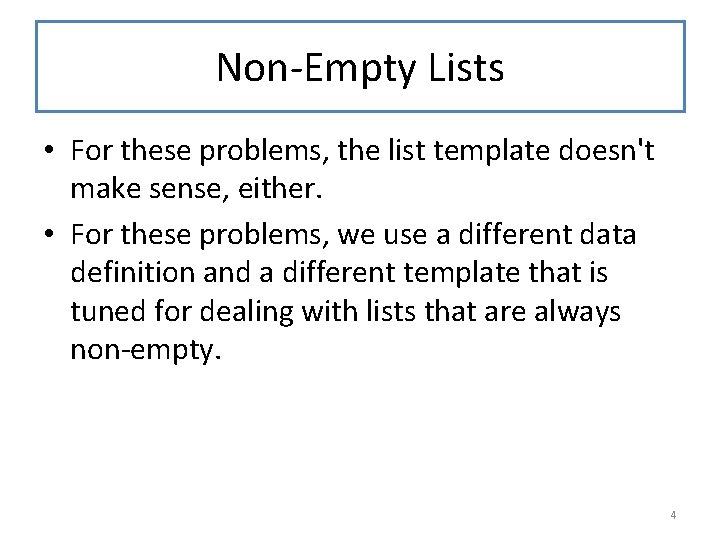Non-Empty Lists • For these problems, the list template doesn't make sense, either. • For these problems, we use a different data definition and a different template that is tuned for dealing with lists that are always non-empty. 4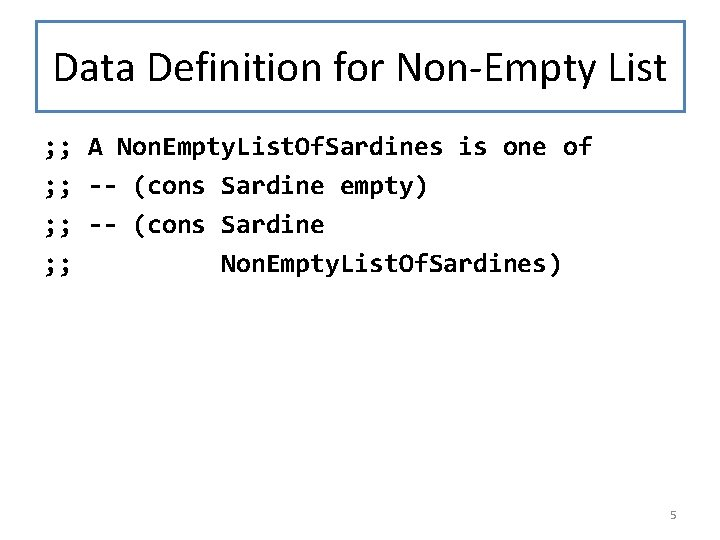Data Definition for Non-Empty List ; ; A Non. Empty. List. Of. Sardines is one of ; ; -- (cons Sardine empty) ; ; -- (cons Sardine ; ; Non. Empty. List. Of. Sardines) 5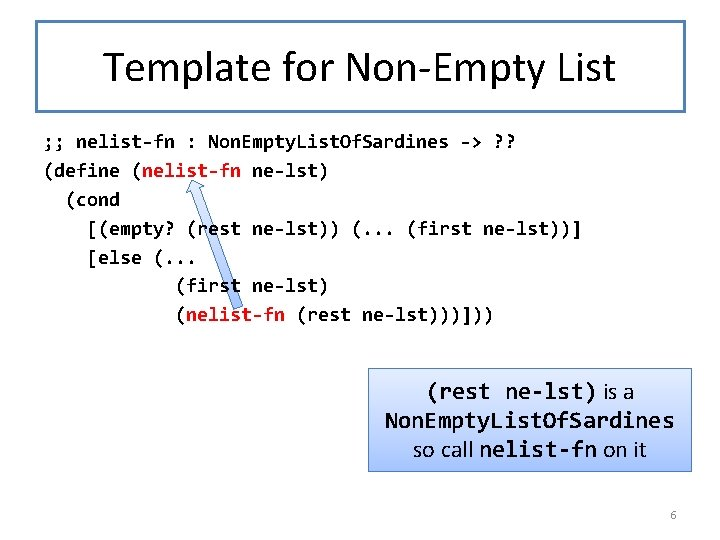Template for Non-Empty List ; ; nelist-fn : Non. Empty. List. Of. Sardines -> ? ? (define (nelist-fn ne-lst) (cond [(empty? (rest ne-lst)) (. . . (first ne-lst))] [else (. . . (first ne-lst) (nelist-fn (rest ne-lst)))])) (rest ne-lst) is a Non. Empty. List. Of. Sardines so call nelist-fn on it 6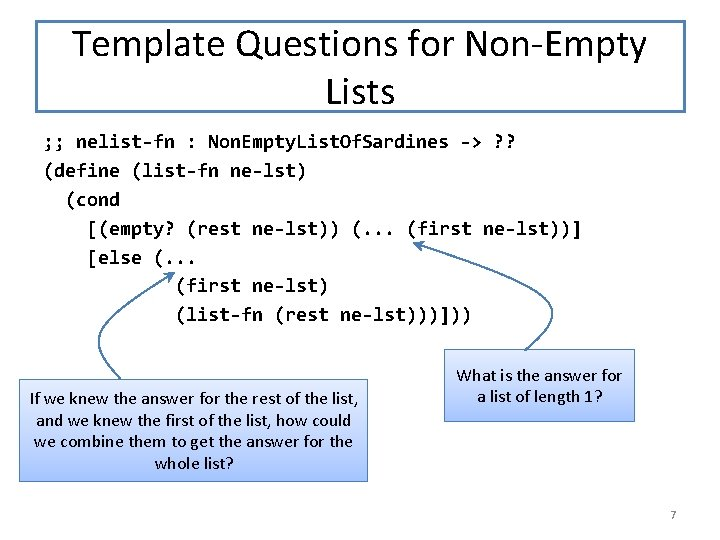Template Questions for Non-Empty Lists ; ; nelist-fn : Non. Empty. List. Of. Sardines -> ? ? (define (list-fn ne-lst) (cond [(empty? (rest ne-lst)) (. . . (first ne-lst))] [else (. . . (first ne-lst) (list-fn (rest ne-lst)))])) If we knew the answer for the rest of the list, and we knew the first of the list, how could we combine them to get the answer for the whole list? What is the answer for a list of length 1? 7Non-Empty Lists: The General Pattern A Non. Empty. List. Of. X is one of -- (cons X empty) interp: a list with a single X -- (cons X Non. Empty. List. Of. X) interp: (cons x lst) represents a sequence whose first element is x and whose other elements are represented by lst. 8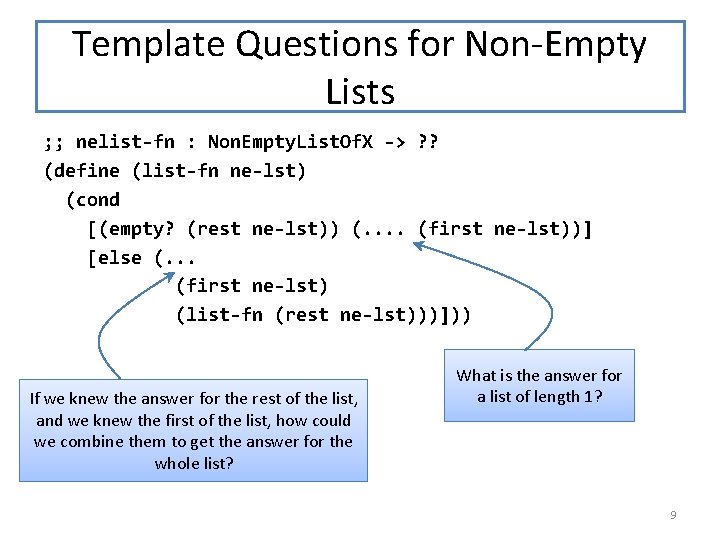Template Questions for Non-Empty Lists ; ; nelist-fn : Non. Empty. List. Of. X -> ? ? (define (list-fn ne-lst) (cond [(empty? (rest ne-lst)) (. . (first ne-lst))] [else (. . . (first ne-lst) (list-fn (rest ne-lst)))])) If we knew the answer for the rest of the list, and we knew the first of the list, how could we combine them to get the answer for the whole list? What is the answer for a list of length 1? 9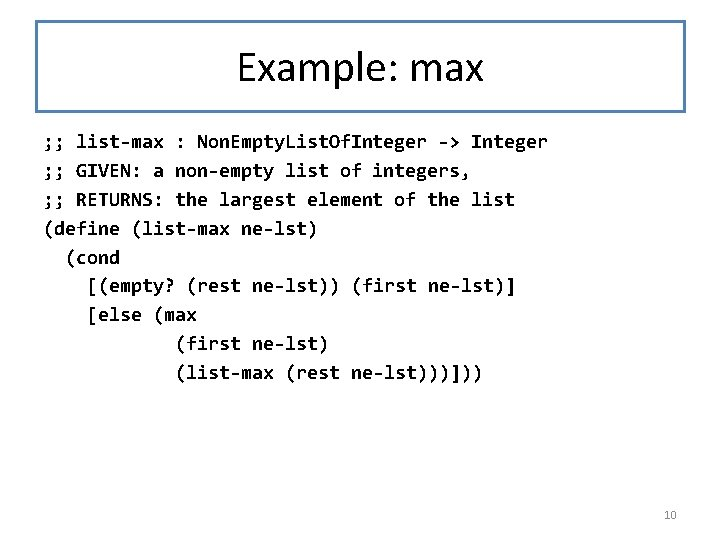Example: max ; ; list-max : Non. Empty. List. Of. Integer -> Integer ; ; GIVEN: a non-empty list of integers, ; ; RETURNS: the largest element of the list (define (list-max ne-lst) (cond [(empty? (rest ne-lst)) (first ne-lst)] [else (max (first ne-lst) (list-max (rest ne-lst)))])) 10Example: average lon-avg : LON -> Number Given a non-empty LON, returns its average (lon-avg (cons 11 empty)) = 11 (lon-avg (cons 33 (cons 11 empty))) = 22 (lon-avg (cons 33 (cons 11 empty)))) = 55/3 11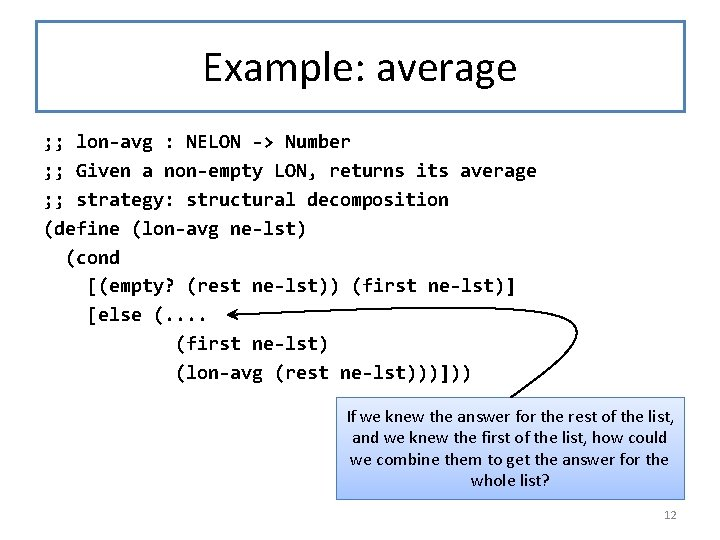Example: average ; ; lon-avg : NELON -> Number ; ; Given a non-empty LON, returns its average ; ; strategy: structural decomposition (define (lon-avg ne-lst) (cond [(empty? (rest ne-lst)) (first ne-lst)] [else (. . (first ne-lst) (lon-avg (rest ne-lst)))])) If we knew the answer for the rest of the list, and we knew the first of the list, how could we combine them to get the answer for the whole list? 12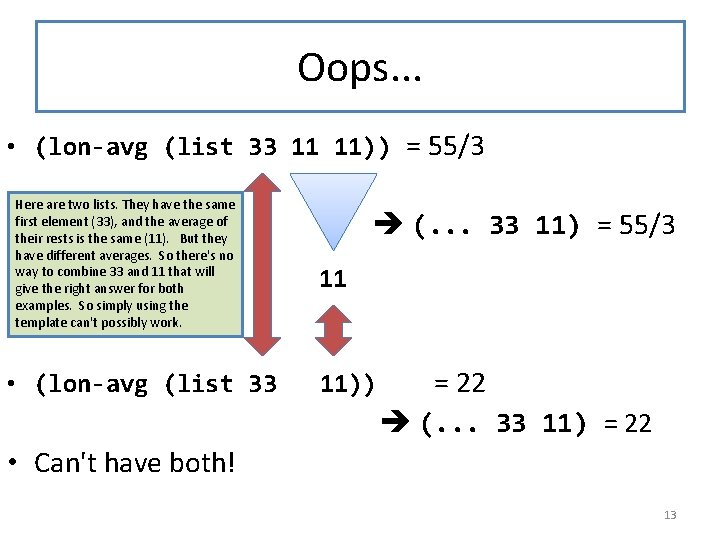Oops. . . • (lon-avg (list 33 11 11)) = 55/3 Here are two lists. They have the same first element (33), and the average of their rests is the same (11). But they have different averages. So there's no way to combine 33 and 11 that will give the right answer for both examples. So simply using the template can't possibly work. • (lon-avg (list 33 (. . . 33 11) = 55/3 11 11)) = 22 (. . . 33 11) = 22 • Can't have both! 13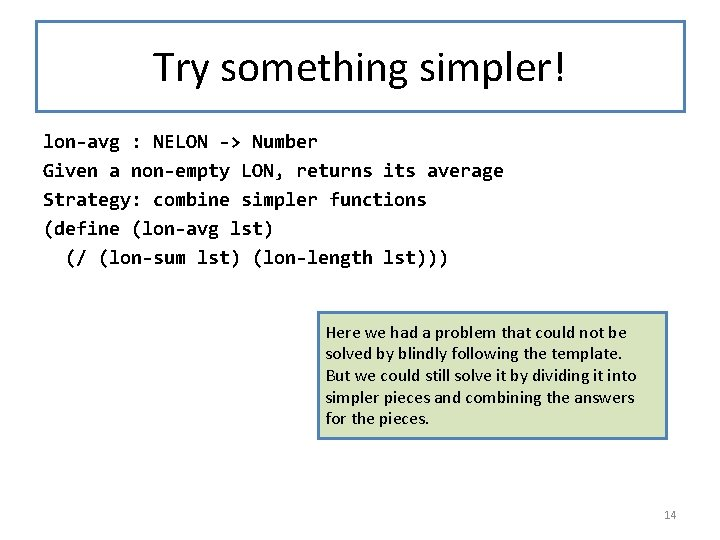Try something simpler! lon-avg : NELON -> Number Given a non-empty LON, returns its average Strategy: combine simpler functions (define (lon-avg lst) (/ (lon-sum lst) (lon-length lst))) Here we had a problem that could not be solved by blindly following the template. But we could still solve it by dividing it into simpler pieces and combining the answers for the pieces. 14Another way of defining non-empty lists A Non. Empty. List. Of. X is a (cons X List. Of. X) 15When to use this one? • Use this one when the first element of the list needs to be treated specially. • This one is most often useful with a help function that takes an X and a List. Of. X's. • We'll see this again in Module 7 when we talk about accumulators and generalizing with invarariants. 16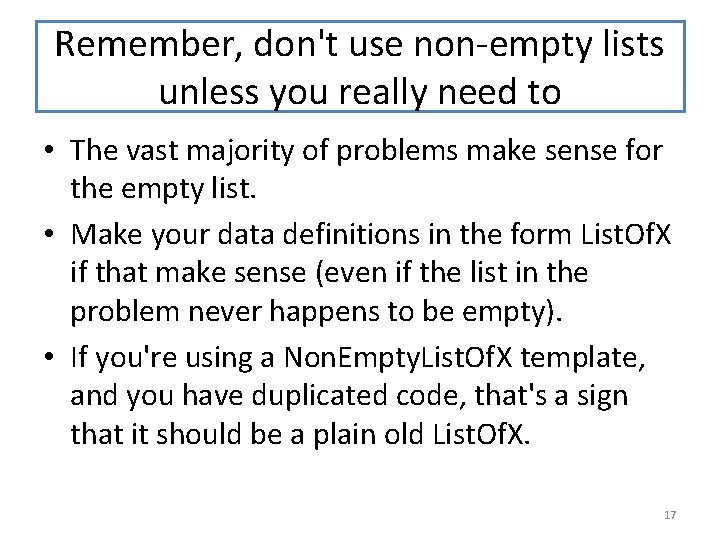Remember, don't use non-empty lists unless you really need to • The vast majority of problems make sense for the empty list. • Make your data definitions in the form List. Of. X if that make sense (even if the list in the problem never happens to be empty). • If you're using a Non. Empty. List. Of. X template, and you have duplicated code, that's a sign that it should be a plain old List. Of. X. 17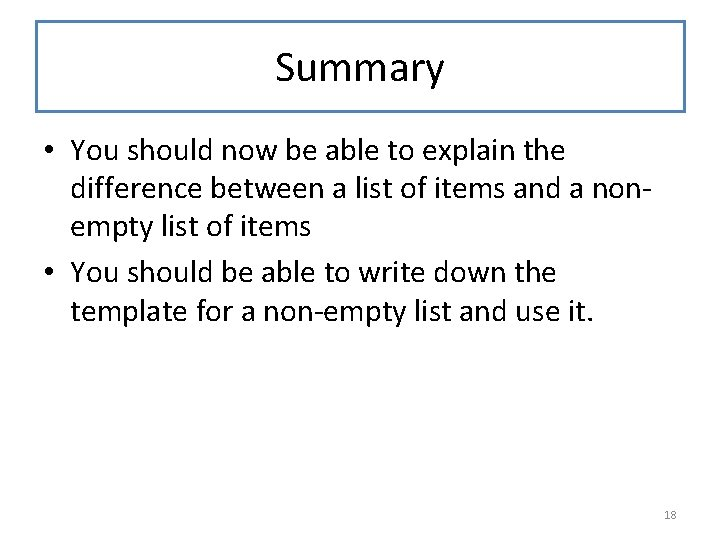Summary • You should now be able to explain the difference between a list of items and a nonempty list of items • You should be able to write down the template for a non-empty list and use it. 18